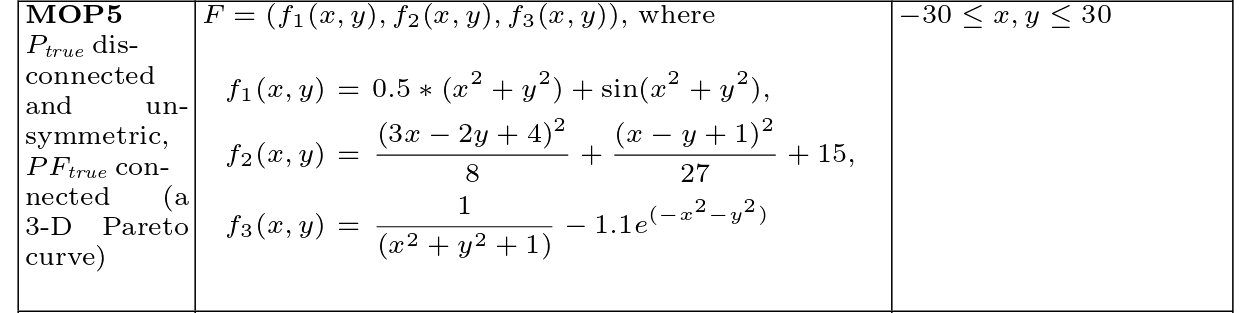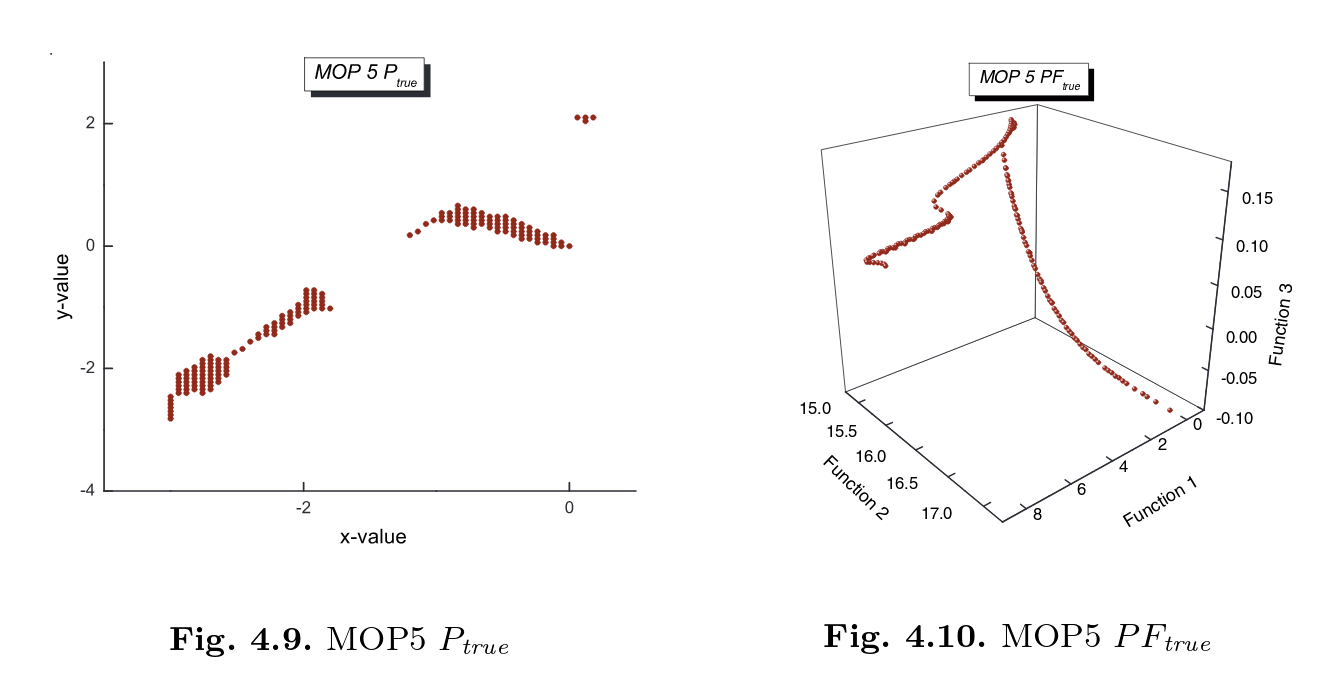# Basics of desdeo-emo

:

import plotly.graph_objects as go
import numpy as np
import pandas as pd

from desdeo_problem import variable_builder, ScalarObjective, MOProblem
from desdeo_problem.testproblems.TestProblems import test_problem_builder

from desdeo_emo.EAs.NSGAIII import NSGAIII
from desdeo_emo.EAs.RVEA import RVEA
from desdeo_emo.utilities.plotlyanimate import animate_init_, animate_next_

from pprint import pprint


## Coello MOP7

### Definition### Pareto set and front## Define objective functions

:

def f_1(x):
term1 = ((x[:,0] - 2) ** 2) / 2
term2 = ((x[:,1] + 1) ** 2) / 13
return term1 + term2 + 3

def f_2(x):
term1 = ((x[:, 0] + x[:, 1] - 3) ** 2) / 36
term2 = ((-x[:, 0] + x[:, 1] + 2) ** 2) / 8
return term1 + term2 - 17

def f_3(x):
term1 = ((x[:, 0] + (2 * x[:, 1]) - 1) ** 2) / 175
term2 = ((-x[:, 0] + 2* x[:, 1]) ** 2) / 17
return term1 + term2 - 13


Note that the expected input x is two dimensional. It should be a 2-D numpy array.

## Create Variable objects

:

help(variable_builder)

Help on function variable_builder in module desdeo_problem.problem.Variable:

variable_builder(names: List[str], initial_values: Union[List[float], numpy.ndarray], lower_bounds: Union[List[float], numpy.ndarray] = None, upper_bounds: Union[List[float], numpy.ndarray] = None) -> List[desdeo_problem.problem.Variable.Variable]
Automatically build all variable objects.

Arguments:
names (List[str]): Names of the variables
initial_values (np.ndarray): Initial values taken by the variables.
lower_bounds (Union[List[float], np.ndarray], optional): Lower bounds of the
variables. If None, it defaults to negative infinity. Defaults to None.
upper_bounds (Union[List[float], np.ndarray], optional): Upper bounds of the
variables. If None, it defaults to positive infinity. Defaults to None.

Raises:
VariableError: Lengths of the input arrays are different.

Returns:
List[Variable]: List of variable objects


:

list_vars = variable_builder(['x', 'y'],
initial_values = [0,0],
lower_bounds=[-400, -400],
upper_bounds=[400, 400])
list_vars

:

[<desdeo_problem.problem.Variable.Variable at 0x18dcf70c340>,
<desdeo_problem.problem.Variable.Variable at 0x18dcf682ca0>]


## Create Objective objects

:

f1 = ScalarObjective(name='f1', evaluator=f_1)
f2 = ScalarObjective(name='f2', evaluator=f_2)
f3 = ScalarObjective(name='f3', evaluator=f_3)
list_objs = [f1, f2, f3]


## Create the problem object

:

problem = MOProblem(variables=list_vars, objectives=list_objs)


## Using the EAs

Pass the problem object to the EA, pass parameters as arguments if required.

:

help(NSGAIII)

Help on class NSGAIII in module desdeo_emo.EAs.NSGAIII:

class NSGAIII(desdeo_emo.EAs.BaseEA.BaseDecompositionEA)
|  NSGAIII(problem: desdeo_problem.problem.Problem.MOProblem, population_size: int = None, population_params: Dict = None, n_survive: int = None, initial_population: desdeo_emo.population.Population.Population = None, lattice_resolution: int = None, selection_type: str = None, interact: bool = False, use_surrogates: bool = False, n_iterations: int = 10, n_gen_per_iter: int = 100, total_function_evaluations: int = 0, keep_archive: bool = False)
|
|  Python Implementation of NSGA-III. Based on the pymoo package.
|
|  Most of the relevant code is contained in the super class. This class just assigns
|  the NSGAIII selection operator to BaseDecompositionEA.
|
|  Parameters
|  ----------
|  problem : MOProblem
|      The problem class object specifying the details of the problem.
|  population_size : int, optional
|      The desired population size, by default None, which sets up a default value
|      of population size depending upon the dimensionaly of the problem.
|  population_params : Dict, optional
|      The parameters for the population class, by default None. See
|      desdeo_emo.population.Population for more details.
|  initial_population : Population, optional
|      An initial population class, by default None. Use this if you want to set up
|      a specific starting population, such as when the output of one EA is to be
|      used as the input of another.
|  lattice_resolution : int, optional
|      The number of divisions along individual axes in the objective space to be
|      used while creating the reference vector lattice by the simplex lattice
|      design. By default None
|  selection_type : str, optional
|      One of ["mean", "optimistic", "robust"]. To be used in data-driven optimization.
|      To be used only with surrogate models which return an "uncertainity" factor.
|      Using "mean" is equivalent to using the mean predicted values from the surrogate
|      models and is the default case.
|      Using "optimistic" results in using (mean - uncertainity) values from the
|      the surrogate models as the predicted value (in case of minimization). It is
|      (mean + uncertainity for maximization).
|      Using "robust" is the opposite of using "optimistic".
|  a_priori : bool, optional
|      A bool variable defining whether a priori preference is to be used or not.
|      By default False
|  interact : bool, optional
|      A bool variable defining whether interactive preference is to be used or
|      not. By default False
|  n_iterations : int, optional
|      The total number of iterations to be run, by default 10. This is not a hard
|      limit and is only used for an internal counter.
|  n_gen_per_iter : int, optional
|      The total number of generations in an iteration to be run, by default 100.
|      This is not a hard limit and is only used for an internal counter.
|  total_function_evaluations :int, optional
|      Set an upper limit to the total number of function evaluations. When set to
|      zero, this argument is ignored and other termination criteria are used.
|
|  Method resolution order:
|      NSGAIII
|      desdeo_emo.EAs.BaseEA.BaseDecompositionEA
|      desdeo_emo.EAs.BaseEA.BaseEA
|      builtins.object
|
|  Methods defined here:
|
|  __init__(self, problem: desdeo_problem.problem.Problem.MOProblem, population_size: int = None, population_params: Dict = None, n_survive: int = None, initial_population: desdeo_emo.population.Population.Population = None, lattice_resolution: int = None, selection_type: str = None, interact: bool = False, use_surrogates: bool = False, n_iterations: int = 10, n_gen_per_iter: int = 100, total_function_evaluations: int = 0, keep_archive: bool = False)
|      Initialize EA here. Set up parameters, create EA specific objects.
|
|  ----------------------------------------------------------------------
|  Methods inherited from desdeo_emo.EAs.BaseEA.BaseDecompositionEA:
|
|  end(self)
|      Conducts non-dominated sorting at the end of the evolution process
|
|      Returns:
|          tuple: The first element is a 2-D array of the decision vectors of the non-dominated solutions.
|              The second element is a 2-D array of the corresponding objective values.
|
|  pre_iteration(self)
|      Run this code before every iteration.
|
|  request_plot(self) -> desdeo_tools.interaction.request.SimplePlotRequest
|
|  request_preferences(self) -> Type[desdeo_tools.interaction.request.BaseRequest]
|
|  requests(self) -> Tuple
|
|  ----------------------------------------------------------------------
|  Methods inherited from desdeo_emo.EAs.BaseEA.BaseEA:
|
|  check_FE_count(self) -> bool
|      Checks whether termination criteria via function evaluation count has been
|          met or not.
|
|      Returns:
|          bool: True is function evaluation count limit NOT met.
|
|  continue_evolution(self) -> bool
|      Checks whether the current iteration should be continued or not.
|
|  continue_iteration(self)
|      Checks whether the current iteration should be continued or not.
|
|  iterate(self, preference=None) -> Tuple
|      Run one iteration of EA.
|
|      One iteration consists of a constant or variable number of
|      generations. This method leaves EA.params unchanged, except the current
|      iteration count and gen count.
|
|  manage_preferences(self, preference=None)
|      Forward the preference to the correct preference handling method.
|
|      Args:
|          preference (_type_, optional): _description_. Defaults to None.
|
|      Raises:
|          eaError: Preference handling not implemented.
|
|  post_iteration(self)
|      Run this code after every iteration.
|
|  set_interaction_type(self, interaction_type: Optional[str]) -> Optional[str]
|
|  start(self)
|      Mimics the structure of the mcdm methods. Returns the request objects from self.retuests().
|
|  ----------------------------------------------------------------------
|  Data descriptors inherited from desdeo_emo.EAs.BaseEA.BaseEA:
|
|  __dict__
|      dictionary for instance variables (if defined)
|
|  __weakref__
|      list of weak references to the object (if defined)
|
|  allowable_interaction_types


:

evolver = NSGAIII(problem,
n_iterations=10,
n_gen_per_iter=100,
population_size=100)

:

while evolver.continue_evolution():
evolver.iterate()


## Extracting optimized decision variables and objective values

:

individuals, solutions, _ = evolver.end()

fig1 = go.Figure(
data=go.Scatter(
x=individuals[:,0],
y=individuals[:,1],
mode="markers"))
fig1